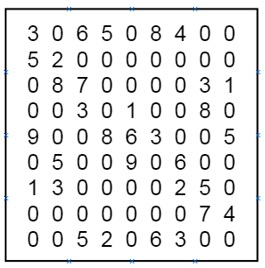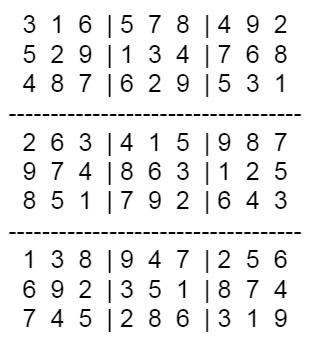# Sudoku Solver in C++

C++Server Side ProgrammingProgramming

Suppose we have a Sudoku grid and we have to solve this famous number maze problem, Sudoku. We know that Sudoku is a 9 x 9 number grid, and the whole grid are also divided into 3 x 3 boxes There are some rules to solve the Sudoku.

• We have to use digits 1 to 9 for solving this problem.

• One digit cannot be repeated in one row, one column or in one 3 x 3 box.

Using backtracking algorithm, we will try to solve Sudoku problem. When some cell is filled with a digit, it checks whether it is valid or not. When it is not valid, it checks for other numbers. If all numbers are checked from 1-9, and no valid digit found to place, it backtracks to previous option.

So if the input is like −The output will be −To solve this, we will follow these steps −

• Define a method called isPresentInCol(), this will take call and num

• for each row r in the grid, do

• if grid[r, col] = num, then return true

• return false otherwise

• Define a method called isPresentInRow(), this will take row and num

• for each column c in the grid, do

• if grid[row, c] = num, then return true

• return false otherwise

• Define a method called isPresentInBox() this will take boxStartRow, boxStartCol, num

• for each row r in boxStartRow to next 3 rows, do

• for each col r in boxStartCol to next 3 columns, do

• if grid[r, c] = num, then return true

• return false otherwise

• Define a method called findEmptyPlace(), this will take row and col

• for each row r in the grid, do

• for each column c in the grid, do

• if grid[r, c] = 0, then return true

• return false

• Define a method called isValidPlace(), this will take row, col, num

• if isPresentInRow(row, num) and isPresentInCol(col, num) and isPresntInBox(row – row mod 3, col – col mod 3, num) all are false, then return true

• Define a method called solveSudoku(), this will take the grid

• if no place in the grid is empty, then return true

• for number 1 to 9, do

• if isValidPlace(row, col, number), then

• grid[row, col] := number

• if solveSudoku = true, then return true

• grid[row, col] := 0

• return false

## Example (C++)

Let us see the following implementation to get a better understanding −

Live Demo

#include <iostream>
#define N 9
using namespace std;
int grid[N][N] = {
{3, 0, 6, 5, 0, 8, 4, 0, 0},
{5, 2, 0, 0, 0, 0, 0, 0, 0},
{0, 8, 7, 0, 0, 0, 0, 3, 1},
{0, 0, 3, 0, 1, 0, 0, 8, 0},
{9, 0, 0, 8, 6, 3, 0, 0, 5},
{0, 5, 0, 0, 9, 0, 6, 0, 0},
{1, 3, 0, 0, 0, 0, 2, 5, 0},
{0, 0, 0, 0, 0, 0, 0, 7, 4},
{0, 0, 5, 2, 0, 6, 3, 0, 0}
};
bool isPresentInCol(int col, int num){ //check whether num is present in col or not
for (int row = 0; row < N; row++)
if (grid[row][col] == num)
return true;
return false;
}
bool isPresentInRow(int row, int num){ //check whether num is present in row or not
for (int col = 0; col < N; col++)
if (grid[row][col] == num)
return true;
return false;
}
bool isPresentInBox(int boxStartRow, int boxStartCol, int num){
//check whether num is present in 3x3 box or not
for (int row = 0; row < 3; row++)
for (int col = 0; col < 3; col++)
if (grid[row+boxStartRow][col+boxStartCol] == num)
return true;
return false;
}
void sudokuGrid(){ //print the sudoku grid after solve
for (int row = 0; row < N; row++){
for (int col = 0; col < N; col++){
if(col == 3 || col == 6)
cout << " | ";
cout << grid[row][col] <<" ";
}
if(row == 2 || row == 5){
cout << endl;
for(int i = 0; i<N; i++)
cout << "---";
}
cout << endl;
}
}
bool findEmptyPlace(int &row, int &col){ //get empty location and update row and column
for (row = 0; row < N; row++)
for (col = 0; col < N; col++)
if (grid[row][col] == 0) //marked with 0 is empty
return true;
return false;
}
bool isValidPlace(int row, int col, int num){
return !isPresentInRow(row, num) && !isPresentInCol(col, num) && !isPresentInBox(row - row%3 ,
col - col%3, num);
}
bool solveSudoku(){
int row, col;
if (!findEmptyPlace(row, col))
return true; //when all places are filled
for (int num = 1; num <= 9; num++){ //valid numbers are 1 - 9
if (isValidPlace(row, col, num)){ //check validation, if yes, put the number in the grid
grid[row][col] = num;
if (solveSudoku()) //recursively go for other rooms in the grid
return true;
grid[row][col] = 0; //turn to unassigned space when conditions are not satisfied
}
}
return false;
}
int main(){
if (solveSudoku() == true)
sudokuGrid();
else
cout << "No solution exists";
}

## Input

{3, 0, 6, 5, 0, 8, 4, 0, 0},
{5, 2, 0, 0, 0, 0, 0, 0, 0},
{0, 8, 7, 0, 0, 0, 0, 3, 1},
{0, 0, 3, 0, 1, 0, 0, 8, 0},
{9, 0, 0, 8, 6, 3, 0, 0, 5},
{0, 5, 0, 0, 9, 0, 6, 0, 0},
{1, 3, 0, 0, 0, 0, 2, 5, 0},
{0, 0, 0, 0, 0, 0, 0, 7, 4},
{0, 0, 5, 2, 0, 6, 3, 0, 0}

## Output

3 1 6 | 5 7 8 | 4 9 2
5 2 9 | 1 3 4 | 7 6 8
4 8 7 | 6 2 9 | 5 3 1
---------------------------
2 6 3 | 4 1 5 | 9 8 7
9 7 4 | 8 6 3 | 1 2 5
8 5 1 | 7 9 2 | 6 4 3
---------------------------
1 3 8 | 9 4 7 | 2 5 6
6 9 2 | 3 5 1 | 8 7 4
7 4 5 | 2 8 6 | 3 1 9